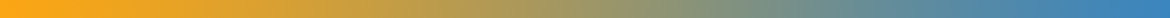### Algorithm

Algorithm basically defines the method of doing some task. In context of software, this term is used to describe the programming logic of a function. Most important factors in deciding the usability of an algorithm are its efficiency of processing and accuracy of the results.

Algorithm are of two types broadly : definitive and subjective. Definite algorithms perform those tasks which have some specific answer whereas subjective algorithms are used when there is no definite answer but matter of opinion is important. Example of a definite algorithm is an algorithm which searches for a word in a list whereas the well known example of subjective algorithms are the algorithms used by public search engines. Each search engine uses a different subjective algorithm to determine that which website best matches your queries.